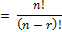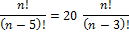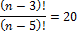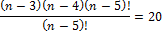# TARGET SBI EXAM 2018: PERMUTATION & COMBINATION

Dear Bankersdaily Aspirants,

Hope , Your preparation for SBI Clerk & PO exam 2018 is good . Since SBI Clerk & PO exam is a Two level which will be conducted in an online mode, Competition will be higher for this SBI Clerk & PO Exam and the most important thing , Current affairs section will be there in this exam. So aspirants have to concentrate in Four sections namely Aptitude, Reasoning, English, Current Affairs.

The person with high speed and very good accuracy only can crack this exam to taste the success. The candidates will be selected on the basis of performance in this online exam test. So the candidate with consistent hard work and regular practice will crack the exam very easily.

Exam: SBI  Clerk & PO Exam 2018

Topic: Permutation & Combination

Day: 25

Timing: 7 minutes

1) Find the value of 9P3.

(a) 504

(b) 309

(c) 405

(d) 600

2) Find the value of n, if nP5 = 20 nP3.

(a) 5

(b) 8

(c) 6

(d) 4

3) How many numbers greater than 5000 can be formed with the digits, 3, 5, 7, 8, 9 no digit being repeated?

(a) 216

(b) 126

(c) 512

(d) 252

4) How many 6 digit telephone numbers can be constructed with the digits 0, 1, 2, 3, 4, 5, 6, 7, 8, 9 if each number starts with 35 and no digit appears more than once?

(a) 1600

(b) 1680

(c) 900000

(d) 9000

5) How many 6 digit numbers can be formed from the digits 1, 2, 4, 5, 6, 7 (no digit being repeated) which are divisible by 5?

(a) 555

(b) 156

(c) 120

(d) None of these

6) How many even numbers greater than 300 can be formed with the digits 1, 2, 3, 4, 5 no digit being repeated?

(a) 111

(b) 600

(c) 900

(d) None of these

7) In how many ways the letters of the word RAINBOW be arranged?

(a) 5040

(b) 4050

(c) 3040

(d) 8040

8) In the word RAINBOW, how many words begin with R?

(a) 720

(b) 360

(c) 1440

(d) None of these

9) In the word RAINBOW, how many words begin with R and ends with W?

(a) 120

(b) 240

(c) 180

(d) 360

10) In the word RAINBOW, how many words are there in which I and O are never together?

(a) 1440

(b) 720

(c) 3600

(d) None of these

1) (a) 504

9P3 = 9!/6!

Because, nPr= (9 ×8 ×7 ×6!)/6!

= 9 × 8 × 7 = 504

2) (b) 8

nP5 = 20 nP3(n – 3)(n – 4) = 20

n = 8 (n = -1 is inadmissible)

3) (a) 216

 4 4 3 2

= 4 × 4 × 3 × 2 = 96

Thousands place cannot assume 3 since required numbers are greater than 5000.

 5 4 3 2 1

= 5 × 4 × 3 × 2 × 1 = 120

Total required numbers = 96 + 120 = 216.

4) (b) 1680

 8 7 6 5

= 8 × 7 × 6 × 5 = 1680

3 and 5 have been already used, so we have 8 digits for thousands place, then 7 digits for hundreds place, 6 digits for tens digit and remaining 5 digits for unit place.

5) (c) 120

A number is divisible by 5 only if its unit digit is either 5 or 0. So we fix 5 as unit digit and then we fill up the remaining places.

 5 4 3 2 1

= 5 × 4 × 3 × 2 × 1 = 120

Hence, required number is 120.

6) (a) 111

Case 1

 3 3 1

= 3 × 3 × 1 = 9

When 2 is fixed at unit place, we have 3, 4 and 5 i.e., 3 digits for hundreds place and remaining 3 digits (out of 5) for the tens place.

 2 3 1

= 2 × 3 × 1 = 6

When 4 is fixed at unit place, we have 3 and 5 i.e., only two digits for hundreds place and remaining 3 digits for tens place.

Case 2

 4 3 2 2

= 48

Case 3

 4 3 2 1 2

= 48

5 digit numbers = 48

Thus there are total 15 + 48 + 48 = 111 even numbers greater than 300.

7) (a) 5040

There are 7 letters in the word RAINBOW and each letter is used only once. So all the 7 letters can be arranged in 7! Ways.

7! = 7 × 6 × 5 × 4 × 3 × 2 × 1 = 5040

8) (a) 720

If we fix R as initial letter, then we have to arrange only 6 remaining letters.

Hence required number of permutations = 6!

= 6 × 5 × 4 × 3 × 2 × 1 = 720

9) (a) 120

If we fix R and W as the first and last letters then we have to arrange only 5 remaining letters which can be arranged in 5! = 120 ways.

10) (c) 3600

Explanation:

Number of permutations when I and O are together

= 2! × 6! = 1440

Total number of permutations = 7! = 5040

Therefore, number of permutations when I and O are not together

= 5040 – 1440 = 3600

 Day 25 Calendar based/weight & height – 1 Permutation & combination Double fillers

Aspirants can also check the other topics from the SBI CLERK STUDY PLANNER from the link given below. DO bookmark the given link as all the posts will be updated in that page and aspirants can check the daily updates from this page or from the Homepage of Bankersdaily.